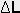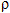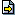Engineering

Horizontal Elliptical

 Show figure Major Axis Position:       Horizontal       Vertical Internal Tank Dimensions: E1 =  m     E2 =  m     c =  m   Level (L) =   m      Length      % Results Memo Volume of the Contents m3 Volume of the Contents (%) % Total Tank Volume m3 Internal Tank Area (Total) m2 Weight of the Contents (1) kgf To  Memory 01 Memory 02 Memory 03 Memory 04 Memory 05 Memory 06 Replacing Adding Subtracting Multiplying Dividing Level Variation ()  m

Notes:

1- The weight of the contents is calculated for a gravitational acceleration equal to 9.80665 m/s2 (sea level)

2- The calculation of the internal area uses one of the formulas for approximation of the ellipse perimeter (Ramanujan - 1914). Therefore, despite presenting a very small error, the total internal area of the tank is not exact

 Configurations Maximum of    decimal places Unit of Measurement of Length:  m      cm      mm      ft      in Specific Weight of the Contents () =   kg/m3Contents:Unit of Measurement of Weight:   kgf g N dyne lbf Impression Page Title (Optional) Comments to be printed (Optional)